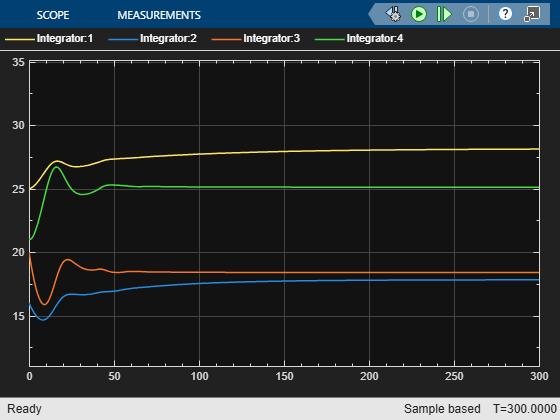# Control of Quadruple-tank Using Passivity-Based Nonlinear MPC

This example shows how to design a passivity-based controller for a quadruple-tank using nonlinear model predictive control (MPC).

### Overview

The dynamics for a quadruple tank system can be written as follows .

`$\stackrel{˙}{\mathit{x}}=\mathit{f}\left(\mathit{x}\right)+\mathit{g}\left(\mathit{x}\right)\mathit{u}$`

where $\mathit{x}$ denotes the heights of the four tanks and $\mathit{u}$ denotes the flows of the two pumps. These dynamics are implemented in `stateFcnQuadrupleTank.m`.

The control objective is to select the flow of the pumps $\mathit{u}$ such that $\left(\mathit{x},\mathit{u}\right)$ moves towards the equilibrium $\left({\mathit{x}}_{\mathit{s}},{\mathit{u}}_{\mathit{s}}\right)$.

To enforce closed-loop stability, the controller includes a passivity constraint.

To define the passivity constraint, first define the state error vector:

`$\mathit{e}=\mathit{x}-{\mathit{x}}_{\mathit{s}}$`

Consider the storage function $\mathit{V}=\frac{1}{2}\left({\mathit{e}}_{3}^{2}+{\mathit{e}}_{4}^{2}\right)$ and take the derivative of $\mathit{V}$ to obtain the relationship 

$\stackrel{˙}{\mathit{V}}\le {{\mathit{u}}_{\mathit{p}}}^{\prime }{\mathit{y}}_{\mathit{p}}$.

This means that, the system is passive from ${\mathit{u}}_{\mathit{p}}=\left[{\mathit{u}}_{2};{\mathit{u}}_{1}\right]-{\mathit{u}}_{\mathit{s}}$ to ${\mathit{y}}_{\mathit{p}}=\left[{\mathit{e}}_{3};{\mathit{e}}_{4}\right]$. The relationship for the passivity input ${\mathit{u}}_{\mathit{p}}$ and the passivity output ${\mathit{y}}_{\mathit{p}}$ are described in the helper function `getPassivityInputQuadrupleTank.m and getPassivityOutputQuadrupleTank.m `.

To enforce closed-loop stability, define the passivity constraint as follows.

${{\mathit{u}}_{\mathit{p}}}^{\prime }{\mathit{y}}_{\mathit{p}}\le -\rho {{\mathit{y}}_{\mathit{p}}}^{\prime }{\mathit{y}}_{\mathit{p}}$ with $\rho >0$.

For a nonlinear MPC controller, you define the passivity constraint by setting the `Passivity` property of the nonlinear MPC object.

### Design Nonlinear MPC Controller

Create a nonlinear MPC object with four states, four outputs, and two inputs.

`nlobj = nlmpc(4,4,2);`
```In standard cost function, zero weights are applied by default to one or more OVs because there are fewer MVs than OVs. ```

Specify the quadruple-tank dynamics function as the state function of the prediction model.

`nlobj.Model.StateFcn = "stateFcnQuadrupleTank";`

The default cost function of a nonlinear MPC problem is a standard quadratic cost function. For this example, keep a quadratic cost function and specify nonzero weights for the first two output variables .

`nlobj.Weights.OutputVariables = [0.1 0.1 0 0];`

Specify the passivity property fields of the nonlinear MPC object.

```nlobj.Passivity.EnforceConstraint = true; nlobj.Passivity.InputFcn = "getPassivityInputQuadrupleTank"; nlobj.Passivity.OutputFcn = "getPassivityOutputQuadrupleTank"; nlobj.Passivity.OutputPassivityIndex = 1;```

### Closed-Loop Simulation

Specify the initial conditions of the states.

`x0 = [25;16;20;21];`

Specify the equilibrium point for the quadruple-tank model.

```xs = [28.1459,17.8230,18.3991,25.1192]'; us = [37,38]';```

```mdl = "quadrupleTankNLMPC"; open_system(mdl)```Run the model.

`sim(mdl);`

View the errors for the quadruple-tank states.

`open_system(mdl + "/Quadruple-tank/state_error")`The errors go to zero and the closed-loop system is stable.

To view the performance of the nonlinear MPC controller without the passivity constraint, remove the passivity constraint from the controller.

`nlobj.Passivity.EnforceConstraint = false;`

Run the simulation.

`sim(mdl);`Without the passivity constraint, the closed-loop system becomes unstable with the same controller design parameters.

### References

 Raff, Tobias, Christian Ebenbauer, and Frank Allgöwer. "Nonlinear model predictive control: A passivity-based approach." In Assessment and Future Directions of Nonlinear Model Predictive Control, edited by Findeisen, Rolf, Frank Allgöwer, and Lorenz T. Biegler, 151-162; New York: Springer, 2007.